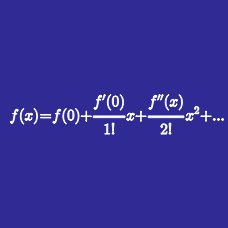Calculus

# Taylor Series - Interval and Radius of Convergence

Which of the following represents the interval of convergence of the Taylor series of $e^x$?

Find the radius of convergence for the series $e^{-3x}$.

Find the radius of convergence for the series $x\sin x$.

Find the radius of convergence for the series $\sqrt{4 + x}$.

Which of the following represents the interval of convergence of the Taylor series of $\ln(1-x)$?

×